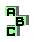CIRCUMCIRCLE    (6/13)

 r597 orthocenter r615 orthopole, Simson line, Steiner deltoid r616 orthopole, orthocenter, altitude feet r619 orthopole, circumcenter, Prasolov point r633 Fuhrmann triangle r642 excircles, ninecircle, point X(650), excenters, tangent circles, radical center r644 point X(110), circumconic, Lemoine line, De Longchamps line, Steiner point, isogonal lines, isotomic lines r661 perspective, isogonal of the infinite point r677 Simson line, Spieker point r680 second Brocard triangle, Brocard circle, circumcenter, Apollonian circles, inverse points r687 Johnson triangle, perspective, symmedian point r697 centroid, orthocenter, circumcenter, symmedian point, point X(110), point X(842) r700 point X(842), point X(681) r702 point X(681), centroid, circumcenter, symmedian point, Parry point r714 ninecenter, circumcenter, point X(186), far-out point, inverse points r717 point X(92), altitudes, tripolar r734 point X(55), antiorthic axis r746 Brocard circle, isodynamic points, inverse points r762 Morley triangle, circumtangential triangle, isotomic points, Steiner circumellipse, third Brocard point, Steiner point, homothetic triangles r767 isogonal points, incenter, point X(80), inverse points r771 circumtangential triangle, anticomplement, Steiner deltoid r781 circumconic, perspector, symmedian point, tripole1 2 3 4 5 6 7 8 9 10 11 12 13